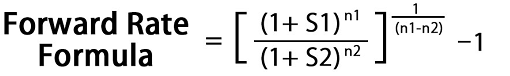#Forward Rate (FR) Calculator

This online tool is used to calculate the forward rate for (n1 - n2) number of years after n2 number of years.

# Results...

Forward Rate = [ (1 + S1)n1 / (1 + S2)n2 ]1/(n1-n2) - 1

Forward Rate = [ (1 + 7.5)2 / (1 + 6.5)1 ]1/(2 - 1) - 1

Forward Rate = [ (8.5)2 / (7.5)1 ]1/(1) - 1

Forward Rate = [ (72.25 / 7.5) ]1 - 1

Forward Rate = [9.633]1 - 1

Forward Rate = 9.633 - 1

Forward Rate = 8.633%## How to calculate forward rate(FR%) :

The forward rate formula helps in deciphering the yield curve which is a graphical representation of yields on different bonds having different maturity periods. It can be calculated based on spot rate on the further future date and a closer future date and the number of years until the further future date and closer future date.

Formula :Where,

FR - Forward Rate.

S1 - Spot rate until a further future date

S2 - Spot rate until a closer future date

n1 - Number of years until a further future date

n2 - Number of years until a closer future date###Recommended Pages ►

Free Cash Flow Calculator